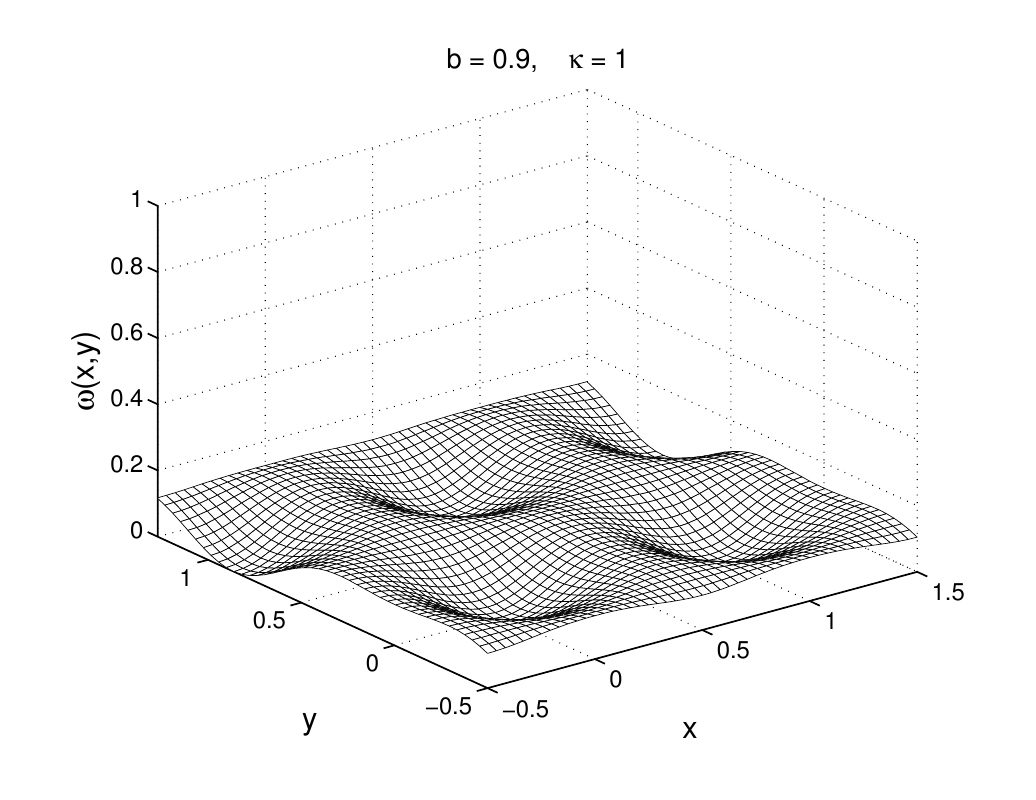# Program calculating vortex lattice

MATLAB program computing the ideal vortex lattice and showing how it looks like can be downloaded here:

## program VL15.zip

 This program VL15.m computes the ideal vortex lattice from GL theory for many kappa and b values. The present version computes only one case kappa = 2, but by omitting comment % in some line one has also a set of many kappa (loops for b=B/Bc2 and kappa) and obtains many magnetization curves. The program shows many plots, most are shown p1 sec long, I put here p1=0.9 seconds, is too short, but then the computation of the mag.curves is faster. This program VL15.m generates two big files fgA.ps and fgB.ps, which contain the 3D plots of \omega(x,y) and B(x,y) and also the magn.curves M(H). Before the run these 2 files should be erased, else these many plots add to them. They get a length 5.75 MB each. If transformed into pdf files (each 3.6 MB), they show nice movies by typing arrow left or arrow right. From this program one may easily make one that shows only one example (b, kappa) but shows the intermediate plots much longer, e.g., p1 = 3 seconds.Earlier version of the VL program can be downloaded here. Here you can see how with changing reduced induction b=B/Bc2 vortex lattice structure changes. The length unit is the vortex spacing, GL parameter κ = 1.